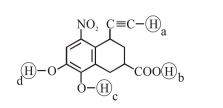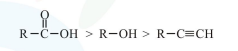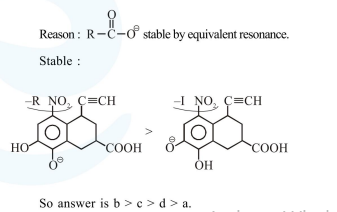# Arrange the following labelled hydrogens

Question:

Arrange the following labelled hydrogens in decreasing order of acidity :1. $b>c>d>a$

2. $\mathrm{c}>\mathrm{b}>\mathrm{a}>\mathrm{d}$

3. $b>a>c>d$

4. $c>b>d>a$

Correct Option: 1

Solution:

Acidic strength order :